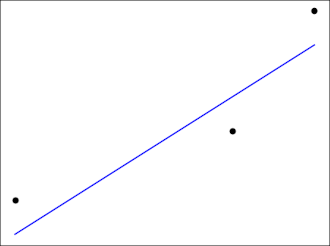# Best line to fit three points

Suppose you want to fit a line to three data points. If no line passes exactly through your points, what’s the best compromise you could make?Chebyshev suggested the best thing to do is find the minmax line, the line that minimizes the maximum error. That is, for each candidate line, find the vertical distance from each point to the line, and take the largest of these three distances as the measure of how well the line fits. The best line is the that minimizes this measure.

Note that this is not the same line you would get if you did a linear regression. Customary linear regression minimizes the average squared vertical distance from each point to the line. There are reasons this is the standard approach when you have at least a moderate amount of data, but when you only have three data points, it makes sense to use the minmax approach.

We can say several things about the minmax line. For one thing, there exists such a line and it is unique. Also, the vertical distance from each data point to the line will be the same. Either two points will be over the line and one under, or two will be under and one over.

Suppose your three points are (x1, y1), (x2, y2), and (x3, y3), with x1 < x2 < x3. Then the slope will beand the intercept will beI made an online calculator to find the best line for three points.

## 4 thoughts on “Best line to fit three points”

1. With no application of the result in mind, I would suggest the lowest average perpendicular distance to the line. Which method produces that?

2. That’s called Deming regression, finding a least-squares fit with orthogonal distance rather than vertical distance.

With the minmax fit, I think minimizing vertical distance and minimizing orthogonal distance both give you the same line. All the vertical distances are the same, so all the orthogonal distances are the same.

3. So if I am fitting lines to 3 points, how much better would the minmax fits be vs the standard regression (ssr) fits? Is it a meaningful difference? And how would I quantify it? And under what circumstances? How does that scale with the number of data points. I’m an experimentalist, and I’ve found ‘better’ is not always worth pursuing at the expense of simplicity (in this case blindly using standard regression).

If I find myself in a circumstance where I am fitting lines to a few points I might do some simulations to think about this more. Thanks!

4. Andreas

We need to clarify terms: linear regression is of the form y=a+b*x, a first-order polynomial.
“Customary linear regression” is commonly known as ordinary least squares (OLS) linear regression.

There are other variants such as L1 linear regression, minimizing the sum of absolute differences, which has its own merits and is less sensitive to (single) extreme values.

Economists relate this to the minimax approach, minimizing the maximum loss.
Better, as usual, is in the eye of the beholder.

Thoughts on this approach:
Two points with identical x-values, e.g., x2=x3, should still allow for estimation of intercept and slope.
Three identical x-values should still allow identifying the “best” (x, yhat) at x=x1=x2=x3, but intercept and slope are arbitrary or not identifiable.